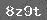# 金菊教育1-6年级数学天天练（2020-02-04）56+39=                  83-26=

200分米=（   ）米           500厘米=（   ）分米
4000米=（   ）千米         2800分米=（   ）米
2千米=（   ）米               1700厘米=（   ）米
3千米-500米=（   ）米    2千米300米=（   ）米
3吨=（   ）千克               5000千克=（   ）吨

301×20=                 512×17=

8.726×59.1=87.26×（）=872.6×（）=0.8726×（）
30名同学按身高由低到高排成一队，相邻两同学的身高差都相同。前10名同学的身高和是12.5米，前20名同学的身高和是26.5米，那么这30名同学的身高和是多少米？

3年前父子的年龄和是49岁，今年父亲的年龄是儿子年龄的4倍，父子今年各多少岁？16-10=6$个$①花5角钱买5个练习本。
②无法回答。因为在路上捡钱是偶然的，人数多不一定能多捡到钱。

200分米=（20）米        500厘米=（50）分米
4000米=（ 4）千米        2800分米=（280）米
2千米=（2000）米        1700厘米=（17）米
3千米-500米=（2500）米  2千米300米=（2300）米
3吨=（3000）千克         5000千克=（5）吨

$1$把木料锯成5段，要锯几次？5-1=4$次$
$2$一共要锯多少分钟？4×3=12$分钟$[$0.5×2+0.5$×2+0.5]×2
=$1.5×2+0.5$×2
=3.5×2=7$个$

5.91   0.591   591
【答案】42米【答案】

### 相关推荐

 留言与评论（共有 0 条评论）

 验证码：